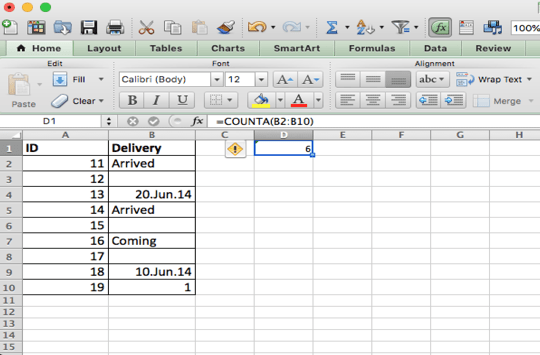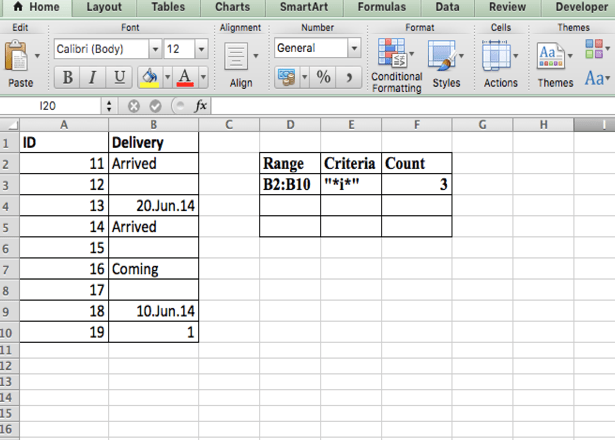Get instant live expert help with Excel or Google Sheets“My Excelchat expert helped me in less than 20 minutes, saving me what would have been 5 hours of work!”

#### Post your problem and you’ll get expert help in seconds.

Your message must be at least 40 characters
Our professional experts are available now. Your privacy is guaranteed.

# Learn How to Apply a Formula for Counting Cells that are Not Blank

Sometimes you have a set of data in Excel that contains some blank cells. You can use the COUNTA function to create a formula that will only count the cells that are not blank.

### COUNTA to Count Cells that are Not Blank

Let’s say you want to count cells that contain data, for example, the numbers of students in a data range. And you would like to ignore any cell that is blank. The way to do go about doing this is to use the COUNTA function.

The COUNTA function allows you to count cells containing any type of information. It can be error values and empty text (“”). If the range contains a formula that returns an empty string, this function counts that value. So the COUNTA function counts all cells that contain any type of “value” and does not count empty cells.

To count the total number of students in a range A1:A30 and add the result in A31 cell then select A31 cell and type :

`=COUNTA(A1:A30)`And press Enter. And the answer will be 6.

### Count Cells Containing Text Values

In case you need to count only cells containing any TEXT VALUES, so excluding cells dates and numbers, treating as blank cells and not including in the count value you can use this formula :

`=COUNTIF (range, “*”)`

The above formula counts only cells containing text values, so that means that cells with dates and numbers will not be included in the count but will be treated as blank cells.

In the generic form of the formula range is a range of cells, and “*” is a wildcard matching any number of text characters.

The formula for counting all non-blank cells in a range is (as COUNTA function above) :

`=COUNTIF (range,”<>”&””)`

This formula will correctly count all type of values – text, dates, and numbers, as you can see in the screenshot below.

### Count Cells Containing Specific Text

To count the number of cells that contain specific text in a range you can use :

`=COUNTIF(range,”*text*”)`So the result in cell F3, shows the counting value in range B2:B10 searching for criteria “i”.

Still need some help with Excel formatting or have other questions about Excel? Connect with a live Excel expert here for some 1 on 1 help. Your first session is always free.

### Did this post not answer your question? Get a solution from connecting with the expert.Another blog reader asked this question today on Excelchat:
Related blogs
Solution examplesI need a formula to count the number of rows that contain at least one of two possible phrases, but not give me the total number of instances that both phrases occur overall.
Solved by E. B. in 33 minsGood morning, I need a cell formatting solution to display leading zero in my excel table with the following conditions. 1. Cannot format as text 2. cell size is not fixed. Please let me know if there is a solution.Thanks in advance
Solved by V. C. in 11 minsi have a table with Dates, First names , Last names and i want to count how many times a name occurs, but if a name occurs more than once on a particular date i need to only count it once. this is on excel and not google sheets
Solved by F. H. in 31 minsNeed a formula that counts (1,2,3,4 ect..in cell M21) a range of cells, N4:N20, which are annual premium values. But I only want to add a sale count if the average monthly premium is above \$30 per sale. So if someone has 4 sales but they don't equal a total of \$120/mth (\$30 x 4 = \$120)... they would only get a count of 3 sales.
Solved by S. E. in 60 minsHello, I am trying to create a total color column? Can anyone help?
Solved by I. A. in 60 mins## Subscribe to Excelchat.coAnother blog reader asked this question today on Excelchat: